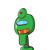# Given a line segment AB. Divide it in the ratio m:n by construction where both m and n are positive integers and let m equals to 4

Given a line segment AB. Divide it in the ratio m:n by construction where both m and n are positive integers and let m equals to 4 and n equals to 3.
IN THIS QUESTION CAN ANYONE TELL, “WHAT IS THE LENGTH OF THE LINE SEGMENT AB; (EITHER 7cm OR SOMETHING ELSE)?
[Therefore, there is no need to answer full question]​

### 1 thought on “Given a line segment AB. Divide it in the ratio m:n by construction where both m and n are positive integers and let m equals to 4”

1.